Students can Download Computer Science Chapter 16 Data Visualization Using Pyplot: Line Chart, Pie Chart and Bar Chart Questions and Answers, Notes Pdf, Samacheer Kalvi 12th Computer Science Book Solutions Guide Pdf helps you to revise the complete Tamilnadu State Board New Syllabus and score more marks in your examinations.

## Tamilnadu Samacheer Kalvi 12th Computer Science Solutions Chapter 16 Data Visualization Using Pyplot: Line Chart, Pie Chart and Bar Chart

### Samacheer Kalvi 12th Computer Science Data Visualization Using Pyplot: Line Chart, Pie Chart and Bar ChartText Book Back Questions and Answers

PART – I

Question 1.
Which is a python package used for 2D graphics?
(a) matplotlib.pyplot
(b) matplotlib.pip
(c) matplotlib.numpy
(d) matplotlib.plt
(a) matplotlib.pyplot

Question 2.
Identify the package manager for Python packages, or modules.
(a) Matplotlib
(b) PIP
(c) plt.show( )
(d) python package
(b) PIP

Question 3.
Read the following code: Identify the purpose of this code and choose the right option from the following.
C:\Users\YourName\AppData\Local\Programs\Python\Python36-32\Scripts>pip -version
(a) Check if PIP is Installed
(b) Install PIP
(d) Check PIP version
(d) Check PIP version

Question 4.
Read the following code: Identify the purpose of this code and choose the right option from the following.
(a) List installed packages
(b) list command
(c) Install PIP
(d) packages installed
(a) List installed packages

Question 5.
To install matplotlib, the following function will be typed in your command prompt. Python -m pip install -U pip What does “-U”represents?
(c) removing pip

Question 6.
Observe the output figure. Identify the coding for obtaining this output.(a) import matplotlib.pyplot as pit
plt.plot( [1,2,3], [4,5,1 ])
plt.show( )

(b) import matplotlib.pyplot as pit plt.plot([l,2],[4,5])
plt.show( )

(c) import matplotlib.pyplot as pit
plt.plot([2,3],[5,l])
plt.show( )

(d) import matplotlib.pyplot as pit
plt.plot([l,3],[4,l])
plt.show( )
(a) import matplotlib.pyplot as pit
plt.plot( [1,2,3], [4,5,1 ])
plt.show( )

Question 7.
(a) import matplotlib.pyplot as pit
(b) plt.plot(3,2)
(c) plt.show( )
Identify the output for the above coding
(a)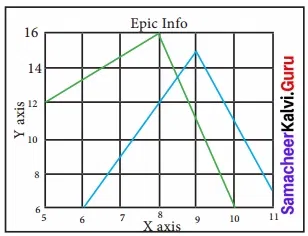(b)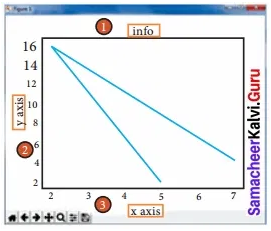(c)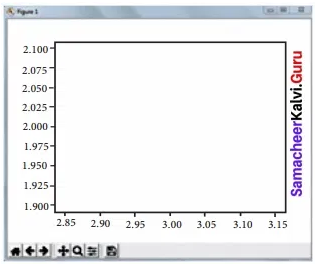(d)(c)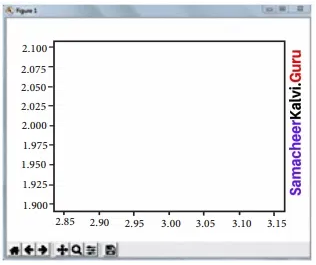Question 8.
Which key is used to run the module?
(a) F6
(b) F4
(c) F3
(d) F5
(d) F5

Question 9.
Identify the right type of chart using the following hints.
Hint 1: This chart is often used to visualize a trend in data over intervals of time.
Hint 2: The line in this type of chart is often drawn chronologically.
(a) Line chart
(b) Bar chart
(c) Pie chart
(d) Scatter plot
(a) Line chart

Question 10.
Read the statements given below. Identify the right option from the following for pie chart.
Statement A: To make a pie chart with Matplotlib, we can use the plt.pie( ) function.
Statement B: The autopct parameter allows us to display the percentage value using the Python string formatting.
(a) Statement A is correct
(b) Statement B is correct
(c) Both the statements are correct
(d) Both the statements are wrong
(c) Both the statements are correct

PART – II

Question 1.
Define: Data Visualization?
Data Visualization is the graphical representation of information and data. The objective of Data Visualization is to communicate information visually to users. For this, data visualization uses statistical graphics. Numerical data may be encoded using dots, lines, or bars, to visually communicate a quantitative message.

Question 2.
List the general types of data visualization?

1. Charts
2. Tables
3. Graphs
4. Maps
5. Infographics
6. Dashboards

Question 3.
List the types of Visualizations in Matplotlib?
There are many types of Visualizations under Matplotlib. Some of them are:

1. Line plot
2. Scatter plot
3. Histogram
4. Box plot
5. Bar chart and
6. Pie chart

Question 4.
How will you install Matplotlib?
We can install matplotlib using pip. Pip is a management software for installing python packages.
After installing Matplotlib, we will begin coding by importing Matplotlib using the command: import matplotlib.pyplot as plt

Question 5.
Write the difference between the following functions: plt.plot([1,2,3,4]), plt.plot([1,2,3,4]), [1.4.9.16] ).
Draw line plt.plot(l,2,3,4] generate x values and given values are y values.
[1.4.9.16]) to plot x verses y

PART – III

Question 1.
Draw the output for the following data visualization plot?
import matplotlib.pyplot as pit
plt.bar([l ,3,5,7,9],[5,2,7,8,2],
label=”Example one”)
plt.bar([2,4,6,8,10],[8,6,2,5,6], label=”Example two”, color=’g’)
pit. legend))
plt.xlabel(‘bar number’)
plt.ylabel(‘bar height’)
pit.title(‘Epic Graph\nAnother Line! Whoa’)
pit. show( )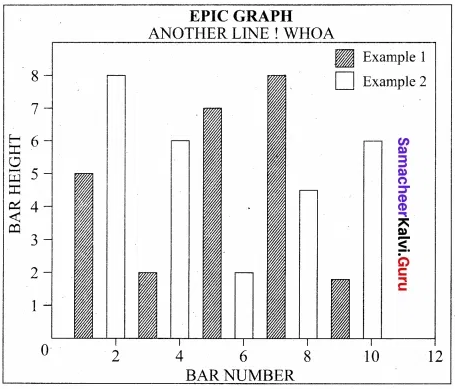Question 2.
Write any three uses of data visualization?

1. Data Visualization help users to analyze and interpret the data easily.
2. It makes complex data understandable and usable.
3. Various Charts in Data Visualization helps to show relationship in the data for one or more variables.

Question 3.
Write the coding for the following:
a. To check if PIP is Installed in your PC.
b. To Check the version of PIP installed in your PC.
c. To list the packages in matplotlib.
In command prompt type pip — version. If it is installed already, you will get version. Python -m pip install – U pip
C:\Users\YourName\AppData\Local\Programs\Python\Python36-32\Scripts>pip – version
C:\Users\YourName\AppData\Local\Programs\Python\Python36-32\Scripts>pip list

Question 4.
Write the plot for the following pie chart output?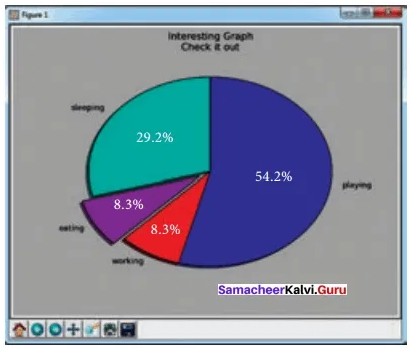‘import matplotlib.pyplot as pit
slices = [7,2,2,13]
activities = [‘sleeping’, ‘eating’, ‘working’, ‘playing’]
plt.pie(slices, labels = activities, autopct = ‘y.1.1 f % % ‘)
plt.title(‘Interesting Graph check it out’)
plt.show( )

PART – IV

Question 1.
Explain in detail the types of pyplots using Matplotlib?
Line Chart:
A Line Chart or Line Graph is a type of chart which displays information as a series of data points called ‘markers’ connected by straight line segments. A Line Chart is often used to visualize a trend in data over intervals of time – a time series – thus the line is often drawn chronologically.
Example: Line plot
import matplotlib.pyplot as plt
years= [2014,2015,2016,2017,2018]
totaljopulations = [8939007, 8954518,8960387,8956741, 8943721]
plt.plot (years, total_populations)
plt.title (“Year vs Population in India”)
plt.xlabel (“Year”)
plt.ylabel (“Total Population”)
plt.legend( )
pit. show( )
In this program,
Plt.title( ) → specifies title to the graph
Plt.xlabel( ) → specifies label for X-axis
Plt.ylabel( ) → specifies label for Y-axis
Output 4Bar Chart
A BarPlot (or BarChart) is one of the most common type of plot. It shows the relationship between a numerical variable and a categorical variable.
Bar chart represents categorical data with rectangular bars. Each bar has a height corresponds to the value it represents. The bars can be plotted vertically or horizontally. It’s useful when we want to compare a given numeric value on different categories. To make a bar chart with Matplotlib, we can use the plt.bar( ) function.
The above code represents the following:
Labels → Specifies labels for the bars.
Usage → Assign values to the labels specified.
Xticks → Display the tick marks along the x-axis at the values represented. Then specify the label for each tick mark.
Range → Create sequence of numbers.

Pie Chart:
Pie Chart is probably one of the most common type of chart. It is a circular graphic which is divided into slices to illustrate numerical proportion. The point of a pie chart is to show the relationship of parts out of a whole.
To make a Pie Chart with Matplotlib, we can use theplt.pie( ) function. The autopct parameter allows us to display the percentage value using the Python string formatting.

Question 2.
Explain the various buttons in a matplotlib window?Home Button → The Home Button will help once you have begun navigating your chart. If you ever want to return back to the original view, you can click on this.
Forward/Back buttons → These buttons can be used like the Forward and Back buttons in your browser. You can click these to move back to the previous point you were at, or forward again.
Pan Axis → This cross-looking button allows you to click it, and then click and drag your graph around.
Zoom → The Zoom button lets you click on it, then click and drag a square that you would like to zoom into specifically. Zooming in will require a left click and drag. You can alternatively zoom out with a right click and drag.
Configure Subplots → This button allows you to configure various spacing options with your figure and plot.
Save Figure → This button will allow you to save your figure in various forms.

Question 3.
Explain the purpose of the following functions:
a. plt.xlabel
b. plt.ylabel
c. plt.title
d. plt.legend( )
e. plt.show( )
After installing Matplotlib, we will begin coding by importing Matplotlib using the command: import matplotlib.pyplot as pit
Now you have imported Matplotlib in your workspace. You need to display the plots. Using Matplotlib from within a Python script, you have to add plt.show( ) method inside the file to display your plot.
With plt.xlabel and plt.ylabel, you can assign labels to those respective axis. Next, you can assign the plot’s title with plt.title, and then you can invoke the default legend with plt
legend( ).
plt.plot (years, total_populations)
plt.title → (“Year vs Population in India”)
plt.xlabel → (“Year”)
plt.ylabel (“Total Population”)
plt.legend( )
plt.show( )
Plt.title( ) → specifies title to the graph
Plt.xlabel( ) → specifies label for X-axis
Plt.ylabel( ) → specifies label for Y-axis

Practice Programs

Question 1.
Create a plot. Set the title, the x and y labels for both axes?
import matplotlib.pyplot as plt
x = [1, 2, 3]
y = [5, 7, 4]
plt.plot(x,y)
plt.xlabel(‘x-axis’)
plt.ylabel(‘y-axis’)
plt.title(‘LINE GRAPH’)
plt.show( )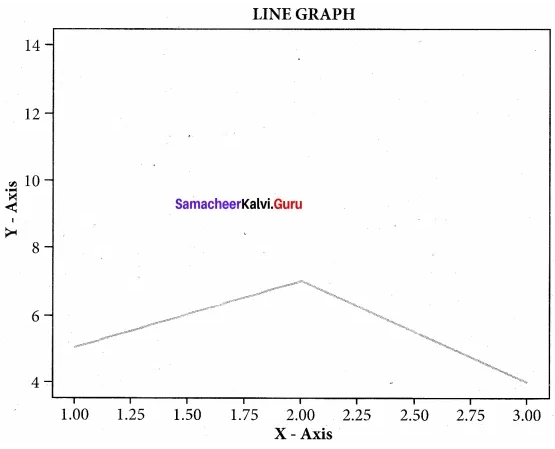Question 2.
Plot a pie chart for your marks in the recent examination?
Example
import matplotlib.pyplot as plt
sizes= [89, 80, 90, 100, 75]
labels= [“Tamil”, “English”, “Maths”, “Science”, “Social”]
plt.pie (sizes, labels Mabels, autopct = “%.2f”)
plt.axes( ).set_aspect (“equal”) plt.sbow( )
Output:Question 3.
Plot a line chart on the academic performance of Class 12 students in Computer Science for the past 10 years?
import matplotlib.pyplot as plt
years = [2009, 2010, 2011, 2012, 2013, 2014, 2015, 2016, 2017, 2018]
cs = [65, 70, 75, 76, 78, 80, 82, 85, 87, 92]
plt.plot(years,cs)
plt.xlabel(“cs”)
plt.ylabel(“years”)
plt.show( )Question 4.
Plot a bar chart for the number of computer science periods in a week?
import matplotlib.pyplot as pit
labels = [“MON”, “TUE”, “WED”, “THUR”, “FRI”, “SAT”, ]
usage = [3, 2, 1,3,2, 2]
y_positions = range(len(labels))
plt.bar(y_positions,usage)
plt.xticks(y_positions, labels)
plt.ylabel(“PERIODS”)
plt.ylabel(“years”)
plt.title(“NO. OF CS PERIODS”)
plt.show( )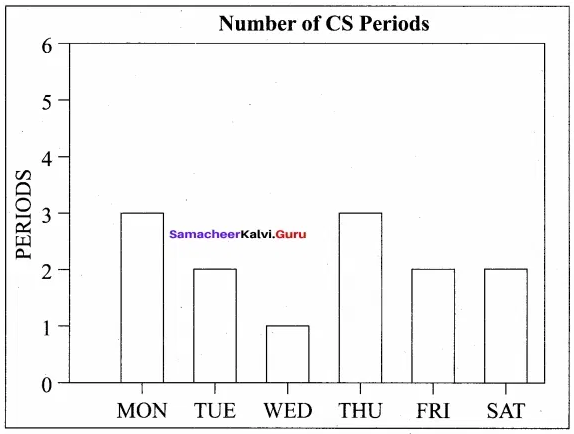### Samacheer kalvi 12th Computer Science Data Manipulation Through SQL Additional Questions and Answers

PART – I

Question 1.
……………………….. is the graphical representation of information and data.
Data Visualization

Question 2.
Pick the odd one out.
Tables, databases, Maps, Infographics, Dashboards
Databases

Question 3.
The representation of information in a graphic format is called …………………………
(a) chart
(b) graphics
(c) Infographics
(d) graphs
Infographics

Question 4.
Read the statements given below. Identify the right option from the following.
Statement A: Dashboards are used to detect patterns and relationships easily.
Statement B: Dashboard is a type of game.
(a) A is correct
(b) B is correct
(c) Both are correct
(d) Both are wrong
(a) A is correct

Question 5.
Various types of visualizations are available in
(a) stdlib
(b) graphics
(c) library
(d) matplotlib
(d) matplotlib

Question 6.
Find the Incorrect match from the following.
(a) Scatter plot – collection of points
(b) line charts – markers
(c) Box plot – Boxes
(c) Box plot – Boxes

Question 7.
The terms minimum, first quartile, median, third quartile maximum are related to ……………………… type of plot.
(a) Box
(b) scatter
(c) pie
(d) Bar
(a) Box

Question 8.
The position of a point in scatter plot is ……………………. value
(a) ID
(b) 2D
(c) 3D
(d) U shaped
(b) 2D

Question 9.
Which method is used to display the plot?
(a) disp( )
(b) display( )
(c) print( )
(d) show( )
(d) show( )

Question 10.
Find the wrong statement
If single list is given to the plot( ) command, matplotlib assumes
(a) it is as a sequence of x values
(b) sequence of y values
(a) it is as a sequence of x values

Question 11.
……………………….. is a versatile command, which takes an arbitrary number of arguments
plot( )

Question 12.
The name to the x axis in the plot is given by ………………………….
(a) label
(b) x
(c) x label
(d) x axis
(c) x label

Question 13.
Which one of the following is the cross-looking button that allows you to click it, and drag the . graph around?
(a) x axis
(b) y axis
(c) pan axis
(d) plot axis
(c) pan axis

Question 14.
……………………. plot shows the relationship between a numerical variable and a categorical variable.
(a) line
(b) Bar
(c) Scatter
(d) Box
(b) Bar

Question 15.
…………………….. assign values to the labels specified in the bar chart.
(a) usage
(b) label
(c) values
(d) =
(a) usage

Question 16.
……………………… displays the tick marks along the x axis at the values represented in bar chart.
x ticks

Question 17.
A ……………………….. represents the frequency distribution of continuous variables.
histogram

Question 18.
Find the Incorrect statement
(a) Histogram is drawn in such a way that there is gap between the bars.
(b) Histogram represents numerical data
(c) Bar graph shows categorical data
(a) Histogram is drawn in such a way that there is gap between the bars.

Question 19.
Which function shows the percentage value in pie chart?
(a) percent
(b) percentage
(c) slice
(d) auto pet
(d) auto pet

Question 20.
Match the following
1. pie chart – (i) Series of data point
2. Histogram – (ii) Parts out of a whole
3. line chart – (iii) Quartile
4. Box plot – (iv) Freqency distribution
(a) 1-i, 2-ii, 3-iii, 4-iv
(b) 1-iv, 2-iii, 3-ii, 4-i
(c) 1 -ii, 2-iv, 3-i, 4-iii
(d) 1-iv, 2-i, 3-ii, 4-iii
(c) 1 -ii, 2-iv, 3-i, 4-iii

PART – II

Question 1.
What is meant by Infographics?
Infographics → An infographic (information graphic) is the representation of information in a graphic format.

Question 2.
Define Scatter plot?
Scatter plot: A scatter plot is a type of plot that shows the data as a collection of points. The position of a point depends on its two-dimensional value, where each value is a position on either the horizontal or vertical dimension.

Question 3.
What is Box Plot?
Box plot: The box plot is a standardized way of displaying the distribution of data based on the five number summary: minimum, first quartile, median, third quartile, and maximum.

PART – III

Question 1.
Define Dashboard?
Dashboard → A dashboard is a collection of resources assembled to create a single unified visual display. Data visualizations and dashboards translate complex ideas and concepts into a simple visual format. Patterns and relationships that are undetectable in text are detectable at a glance using dashboard.

Question 2.
Write a program to create pie chart?
Example
import matplotlib.pyplot as plt
sizes= [89, 80, 90, 100, 75]
labels= [“Tamil”, “English”, “Maths”, “Science”, “Social”]
plt.pie (sizes, labels Mabels, autopet = “%.2f’)
plt.axes( ).set_aspect (“equal”)
plt.show( )
Output: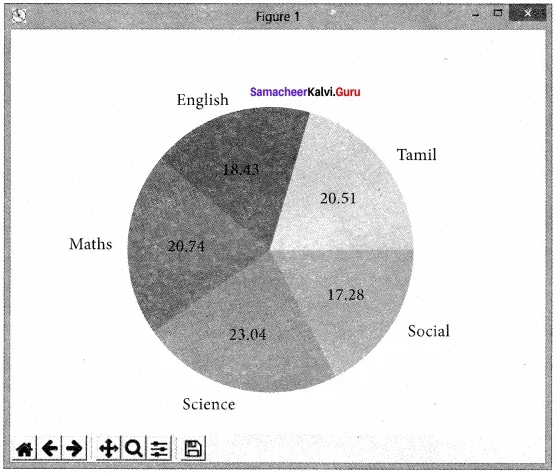PART – IV

Question 1.
Write a program for Bar chart?
Example
import matplotlib.pyplot as pit
#Our data
labels= [“TAMIL”, “ENGLISH”, “MATHS”, “PHYSICS”, “CHEMISTRY”, “CS”]
usage= [79.8, 67.3, 77.8, 68.4, 70.2, 88.5]
# Generating they positions. Later, we’ll use them to replace them with labels.
y_positions =range (len(labels))
#Creating our bar plot plt.bar (y_positions, usage)
plt.xticks (y_positions, labels)
plt.ylabel (“RANGE”)
pit.title (“MARKS”)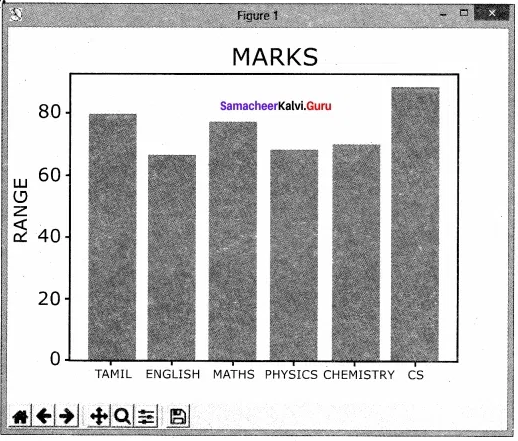Question 2.
Explain the key differences between Histogram and Bar Graph?